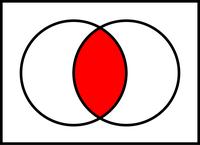# Glossary

## Intersection

The intersection of two sets is the new set formed by taking those elements that are contained in both sets. If $A$ and $B$ are sets, then their intersection is written $A \cap B$. As a result of this definition, $A \cap B$ is a subset of both $A$ and $B$. Compare to the union $A \cup B$, which is the collection of elements contained in either set.

In the standard figure below, two sets are represented by circles (shaded white), and their intersection has been shaded red.